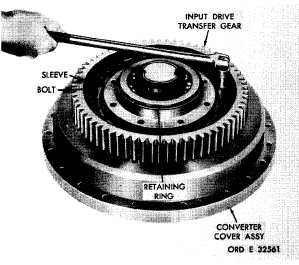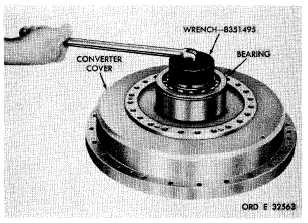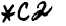Custom SearchP A R    8 2 - 8 3 T O R Q U E     C O N V E R T E R     R E B U I L D S e c t i o n    I V .    T O R Q U E    C O N V E R T E R — R E B U I LD C H A P   5,   SEC  I V 8 2 .     D E S C R I P T I ON Refer   to   p   a   r.   9   for   description   of   the t o r q u e    c o n v e r t e r    a s s e m b l y. (5)  Using   spanner   wrench   8351495,   re- move   the   converter   lock   nut   (fig.   144). (6)   Remove   the   double-row   ball   bearing from   the   hub   of   the   converter   cover. 83. DISASSEMBLY   (fig.   373,   fold-out   2) .   All   related   items   not   covered in     through   ,   below,   were   removed from   the   transmission   as   outlined   in p a r .    7 4 ,    s t e p s    1    t h r o u g h    2 5 .    No f u r t h e r    d i s a s s e m b l y    o f    t h o s e    p a r t s i s    r e q u i r e d. .   T o r q u e    C o n v e r t e r    C o v e r ( 1 )    U s i n g    a    5 / 8 - i n c h    w r e n c h ,    r e m o ve 24   self-locking   bolts   retaining   the   input   drive t r a n s f e r     g e a r     ( f i g .     1 4 2 ) .     R e m o v e     t h e     g e a r. (2)   Remove   the   retaining   ring   from   the torque   converter   support   bearing . (3)   Remove   the   bearing   support   sleeve. ( 4 )    U s i n g    a    h a m m e r    a n d    p u n c h ,    b e nd the   lip   of   the   converter   lock   nut   away   from   the n o t c h e s    i n    t h e    h u b    o f    t h e    t o r q u e    c o n v e r t er cover   (fig.   143). Figure 143. Straightening lip of torque converter lock  nut Figure 142. Removing (or installing) input drive transfer gear Figure 144. Removing (or installing) converter lock nut 1 0 5